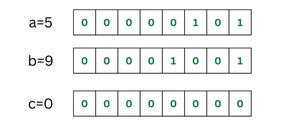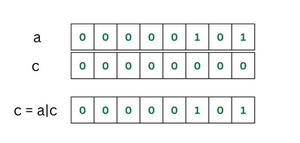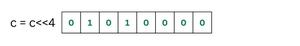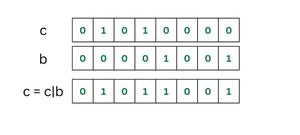Open in App
Not now

# Store two numbers in one Byte using Bit manipulation

• Last Updated : 16 Feb, 2023

Given two values A and B (A, B < 16), the task is to store the numbers in one byte.

Examples:

Input: A = 5, B = 9
Output: 89
Explanation: 5 in binary form is 0101 and the binary form for 9 is 1001. Since 1 byte contains 8 bits, we can store these two nibbles in that 1 bit, forming 01011001 which when converted into decimal becomes 89.

Input: A = 2, B = 10
Output: 42
Explanation: 2 in binary form is 0010 and the binary form for 10 is 1010. Since 1 byte contains 8 bits, which when converted into decimal becomes 42.

Approach: To solve the number follow the below idea:

This problem is much easier to understand by studying the numbers in binary details. As each of the number have maximum 4 bits we can use a nibble to store one number.

Nibble is a four-bit aggregation or half an octet. For 1 byte that is equivalent to 8 bits, it can said that two nibbles make one byte. Now we can use one nibble to represent one number, making it possible to store two numbers in one byte.

Illustration:

Consider:

a = 5 (In binary form a can be written as “00000101”).
b = 9 (In binary form b can be written as “00001001”).
Initially, c = 0 and in binary form 00000000.

For storing a and b in c:The byte representation of the numbers initially

Step 1: c = a | c (where, | is the OR operator)
which will give us “00000101”. We can see the nibble a appear in c.Byte representation of numbers after first step

Step 2: Left shift c by 4 units. This will shift the position of a in c by 4 units to the left.
c = c<<4
c = 01010000Left shift c by 4

Step 3: Now we will add nibble b in c. So perform bitwise OR.
c = c | b
c = 01011001Two numbers stored in a byte

If we convert this binary number c into integer we get c = 89, which we obtained as our output for storing number a and b in one byte of c.

Follow the steps to solve the problem:

• Create an additional bit c to store the final integer value.
• Initially, c is in binary form 00000000.
• Now, OR c with a, as a result, nibble a appear in c (c = a | c).
• Then, left shift the nibble a by 4 units.
• Add nibble b in c by performing OR of b with c  (c = c | b).

Below is the implementation of the above approach.

## C++

 `// C++ program to store two nibble of` `// numbers in a single byte`   `#include ` `using` `namespace` `std;`   `int` `main() {` `int` `a = 5, b = 9, c = 0;` `  ``c = (a << 4) | b;`   `cout << ``"Byte storing two numbers = "` `<< c << endl;`   `return` `0;` `}`   `//This code is contributed by shivamsharma215`

## Java

 `// Java program to store two nibble of` `// numbers in a single byte`   `class` `GFG {`   `    ``// Driver code` `    ``public` `static` `void` `main(String[] args)` `    ``{` `        ``// Numbers to be stored in one byte` `        ``byte` `a = ``5``, b = ``9``;`   `        ``byte` `c = ``0``;`   `        ``// Typecasted to byte` `        ``c = (``byte``)(a << ``4``);`   `        ``// Typecasted to byte` `        ``c = (``byte``)(c | b);`   `        ``System.out.println(``"Byte storing two numbers = "` `                           ``+ c);` `    ``}` `}`

## Python3

 `# Python3 program to store two nibble of` `# numbers in a single byte`   `# Driver code` `if` `__name__ ``=``=` `"__main__"` `:` `    `  `    ``# Numbers to be stored in one byte` `    ``a ``=` `5``; b ``=` `9``;` `    ``c ``=` `0``;`   `    ``# Typecasted to byte` `    ``c ``=` `(a << ``4``);`   `    ``# Typecasted to byte` `    ``c ``=` `(c | b);`   `    ``print``(``"Byte storing two numbers = "``, c);` `    `  `    ``# This code is contributed by AnkThon`

## C#

 `// C# implementation` `using` `System;` `public` `class` `GFG {`   `  ``static` `public` `void` `Main()` `  ``{`   `    ``// Numbers to be stored in one byte` `    ``byte` `a = 5, b = 9;`   `    ``byte` `c = 0;`   `    ``// Typecasted to byte` `    ``c = (``byte``)(a << 4);`   `    ``// Typecasted to byte` `    ``c = (``byte``)(c | b);`   `    ``Console.WriteLine(``"Byte storing two numbers = "` `                      ``+ c);` `  ``}` `}`   `// This code is contributed by ksam24000`

## Javascript

 `// JavaScript program to store two nibble of` `// numbers in a single byte`   `// Driver code` `// Numbers to be stored in one byte` `let a = 5, b = 9;`   `let c = 0;`   `// Typecasted to byte` `c = (a << 4);`   `// Typecasted to byte` `c = (c | b);`   `console.log(`Byte storing two numbers = \${c}`);`   `// This code is contributed by rakeshsahni`

Output

`Byte storing two numbers = 89`

Time Complexity: O(1)
Auxiliary Space: O(1)

Efficient Approach: To solve the problem follow the below steps:

• Now, to store these two nibbles as described above we employ the use of bit merging and shift operators. And to obtain back these two numbers we use bit masking. Both bit masking and bit merging are essential applications of Bit Operators.
• So, we got the number that actually stores two numbers by using bit merging. Now we can obtain back two original stored numbers by using bit masking to see whether our obtained numbers really contain the two stored numbers.

Below is the implementation for the above approach:

## C++

 `// C++ program to store two` `// numbers in a byte` `#include ` `using` `namespace` `std;`   `int` `main()` `{` `    ``// Numbers to be stored in one byte` `    ``char` `a = 5, b = 9;` `    ``char` `c = 0;`   `    ``// bit merging` `    ``c = (``char``)(a << 4); ``// Typecasted to byte` `    ``c = (``char``)(c | b); ``// Typecasted to byte`   `    ``cout << ``"Byte storing two numbers = "` `<< (``int``)c << endl;`   `    ``// Getting back our two stored numbers`   `    ``// Apply bit masking` `    ``cout << ``"first number stored = "` `         ``<< ((c & 0b11110000) >> 4) << endl;` `    ``cout << ``"second number stored = "` `<< (c & 0b00001111)` `         ``<< endl;`   `    ``return` `0;` `}`   `// This Code is Contributed by Prasad Kandekar(prasad264)`

## Java

 `// Java program to store two` `// numbers in a byte`   `class` `GFG {` `    ``public` `static` `void` `main(String[] args)` `    ``{`   `        ``// Numbers to be stored in one byte` `        ``byte` `a = ``5``, b = ``9``;` `        ``byte` `c = ``0``;`   `        ``// bit merging` `        ``c = (``byte``)(a << ``4``); ``// Typecasted to byte` `        ``c = (``byte``)(c | b); ``// Typecasted to byte`   `        ``System.out.println(``"Byte storing two number = "` `                           ``+ c);`   `        ``// Getting back our two stored number`   `        ``// Apply bit maksing` `        ``System.out.println(``"first number stored = "` `                           ``+ ((c & 0b11110000) >> ``4``));` `        ``System.out.println(``"second number stored = "` `                           ``+ (c & 0b00001111));` `    ``}` `}`

## C#

 `// C# program for above approach` `using` `System;` `class` `GFG` `{`   `  ``public` `static` `void` `Main()` `  ``{` `    ``// Numbers to be stored in one byte` `    ``byte` `a = 5, b = 9;` `    ``byte` `c = 0;`   `    ``// bit merging` `    ``c = (``byte``)(a << 4); ``// Typecasted to byte` `    ``c = (``byte``)(c | b); ``// Typecasted to byte`   `    ``Console.WriteLine(``"Byte storing two number = "` `                      ``+ c);`   `    ``// Getting back our two stored number`   `    ``// Apply bit maksing` `    ``Console.WriteLine(``"first number stored = "` `                      ``+ ((c & 0b11110000) >> 4));` `    ``Console.WriteLine(``"second number stored = "` `                      ``+ (c & 0b00001111));` `  ``}` `}`   `// This code is contributed by sanjoy_62.`

## Python3

 `# Python code for the equivalent Java code` `if` `__name__ ``=``=` `'__main__'``:` `  `  `    ``# Numbers to be stored in one byte` `    ``a ``=` `5` `    ``b ``=` `9` `    ``c ``=` `0`   `    ``# Bit merging` `    ``c ``=` `(a << ``4``) & ``0xff` `# Masking to limit the value to a byte` `    ``c ``=` `c | b`   `    ``print``(``"Byte storing two numbers ="``, c)`   `    ``# Getting back our two stored numbers`   `    ``# Apply bit masking` `    ``print``(``"first number stored ="``, (c & ``0b11110000``) >> ``4``)` `    ``print``(``"second number stored ="``, c & ``0b00001111``)`   `# This code is contributed by Edula Vinay Kumar Reddy`

## Javascript

 `// Javascript program to store two` `// numbers in a byte`   `        ``// Numbers to be stored in one byte` `        ``let a = 5, b = 9;` `        ``let c = 0;`   `        ``// bit merging` `        ``c = a << 4; ``// Typecasted to byte` `        ``c = c | b; ``// Typecasted to byte`   `        ``console.log(``"Byte storing two number = "` `                           ``+ c);`   `        ``// Getting back our two stored number`   `        ``// Apply bit maksing` `        ``console.log(``"first number stored = "` `                           ``+ ((c & 0b11110000) >> 4));` `        ``console.log(``"second number stored = "` `                           ``+ (c & 0b00001111));` `    `  `    `  `    ``// This code is contributed by garg28harsh.`

Output

```Byte storing two number = 89
first number stored = 5
second number stored = 9```

Time Complexity: O(1)
Auxiliary Space: O(1)

Related Articles:

My Personal Notes arrow_drop_up
Related Articles